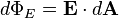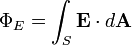Home » Posts tagged 'area vector'

# Tag Archives: area vector

## Electric Flux Misconception

“Could you please tell me if electric flux is defined as no of lines passing through the area then its ans should only be scalar like 5,8,1,6 etc. why its units are given?”

[warning]Electric Flux is a scalar quantity[/warning]

Please note that electric field lines are imaginary lines to represent electric field diagrammatically. And the definition of electric flux in terms of electric lines of force is just a visual aid to understand the concept of electric flux. The actual definition is not in terms of electric lines of force. If it were so, try finding the answers to these questions too and you will realise the problem

1. “If electric flux through a spherical surface around a point charge is one, then you may feel that there is only one line of force. In which direction will it be? By symmetry, there must  be electric field in all directions around a point charge”
2. “What is the electric flux due to the smallest charge existing freely, i.e; the charge of an electron?  When calculated, it comes to be of the order of 2 x 10-8. How can the number of lines be a fraction?

Please see the definintion of electric flux here:

Wikipedia:

“The electric fluxthrough a small areais given by(the electric field, E, multiplied by the component of area perpendicular to the field). The electric flux over a surface S is therefore given by the surface integral:where E is the electric field and dA is a differential area on the closed surface S with an outward facing surface normal defining its direction.”

Hyperphysics:

“The electric flux through a planar area is defined as the electric field times the component of the area perpendicular to the field.”

Watch a video on electric flux here

## A Question from Electric Flux and Gauss Theorem

How can you prove that dS in φ =E.dS is dS Cos θ if the area is tilted at an angle ? I need the mathematical Steps.

Ans;

Dear Zeenath,
Electric Flux is defined as the total no of field lines passing normal to the surface. So while calculating, we need to consider the area of the surface normal to the field lines only. That is why we take the dot product of E and ds, where ds is the area vector (not just the area: Area vector is a vector whose magnitude is equal to the area and dirtected normal to the surface.)
Then by definition of dot product,
dφ =E.dS = EdS cos θ
which gives the component of E and the component of area vector in the direction of E (When area vector is in the direction of E, the actual component of area is perpendicular to E)
Hope the matter is clear.
Mathew Abraham

### Visitors So Far @ AskPhysics

• 2,167,867 hits

### Subscribe to Blog via EmailAsk Physics